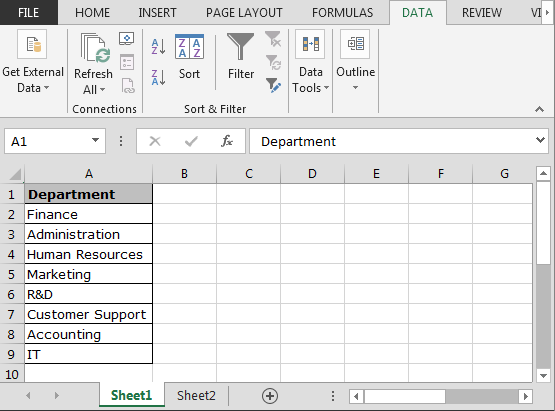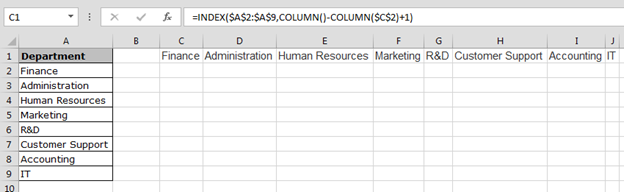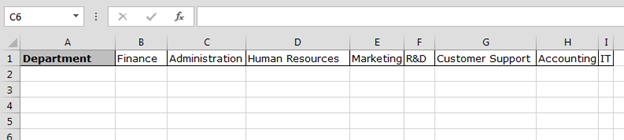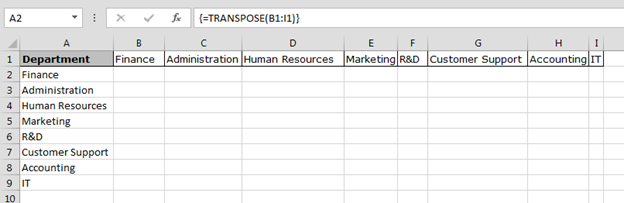# How to Transpose in Excel?

In this article, we will learn how to switch the row value into column value and column value into row value through Transpose formula in Excel.

Problem is: -Transposing the values in List1 (cells A2:A5) into a row, and the values in List2 (cells B10:F10) in to a column.

In Excel, we have Transpose formula to update the column data in row and row data in column. Also, we can use Index function along with Row function to convert the row data in column, and we will use a combination of Index function and column function to convert the column data in row.

Let’s take an example and understand how we can transpose the values:-

To transpose the values in Column A (List1) into a Row:• Enter the formula in cell C1.
• =INDEX(\$A\$2:\$A\$9,COLUMN()-COLUMN(\$C\$2)+1), and press Enter.
• Copy the formula C1:I1.We can use Transpose function. Let’s see how we use the Transpose function.

• Select the range C1:I1 and press F2.
• Enter the formula in cell =TRANSPOSE(A2:A5)
• Press Ctrl+Shift+Enter on your keyboard.How to transpose from a Row into a Column?

We have data in range A1:I1, and we want to update this data in range A2:A9.• Enter the formula in cell A2.
• =INDEX(\$B\$1:\$I\$1,ROW()-ROW(\$K\$1)), and press Enter.
• Copy the formula A2:A9.We can use Transpose function as well. Now, let’s see how we can use the Transpose function.

• Select the range A2:A9 and press F2.
• Enter the formula in cell =TRANSPOSE(B1:I1)
• Press Ctrl+Shift+Enter on your keyboard.This is the way we can update the row data into column and column data into row through formula in Excel.

Below you can find more example to learn about Transpose:-

How to transpose cells in Microsoft Excel

How to use Transpose function

How to Transpose in Microsoft Excel 2007

How to Copy Vertical and Paste Horizontal in Microsoft Excel